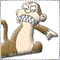# Turning EA on/off at specified times of day regularly.215

Been trying this all day but now just getting myself more and more confused. Say that I want my EA to stop trading from 8:00pm until 6:00am the following day, how would I go about this? I have code for finding the relative GMT offsets for the broker, local time, London, NY etc all working correctly so in what time zone the inputs are isn't a concern. I then wanted to use the function below from TimeSuite.mqh posted on this site (comments added are mine to help aid understanding). Let's assume I the times input are in GMT.

So I have the lines

```   datetime iRangeStart = StrToTime(sRangeStart)+BrokerGMTOffset*3600;
datetime iRangeStop = StrToTime(sRangeStop)+BrokerGMTOffset*3600;```

In situations as above (8:00pm - 6:00am) how do I take into account that 6:00am would convert to be 'less than' 8:00pm once server time passed 00:00 and a new day started? Any and all help would be appreciated, going mad trying to do this.

```int TimeInRange(datetime tTime, string sStart, string sEnd, int iAdjust=15) {

int      iDOW = TimeDayOfWeek(tTime);
//tTime will be current time TimeCurrent
//86400 is number of seconds in a day
//tTime and 86400 are both integers so will return a whole number of full days that have passed.
//Number of hours represented in seconds - StrToInteger(StringSubstr(sStart,2,2))*3600
//Number of minutes represented in seconds - StrToInteger(StringSubstr(sStart,5,2))*60
//iAdjust time can be a negative number for the range to start earlier.
datetime tStart = (tTime/86400)*86400 + (StrToInteger(StringSubstr(sStart,0,1)) - iDOW)*86400
+ StrToInteger(StringSubstr(sStart,2,2))*3600 + StrToInteger(StringSubstr(sStart,5,2))*60

datetime tEnd   = (tTime/86400)*86400 + (StrToInteger(StringSubstr(sEnd,0,1)) - iDOW)*86400
+ StrToInteger(StringSubstr(sEnd,2,2))*3600

if(tTime <= tEnd && tStart > tEnd) {
return(tEnd - tTime);
}

//- Can only be if the 'day' number is less also.
if(tEnd < tStart) {
tEnd = tEnd+86400*7;
}

if(tTime >= tStart && tTime <= tEnd) {
return(tEnd - tTime);
}

return(0);
}
```1758

WBC

Use a function that has two sessions, eg this OTTOMH...

```extern int StartHour.1 = 6;
extern int EndHour.1   = 12;

extern int StartHour.2 = 12;
extern int EndHour.2   = 20;

start()
{
//----
// Do stuff every tick

//----

//----

if (TradingHours() == false) return (0); // Quit here if outside hours

//----

}

{

if ((Hour()>= StartHour.1) && (Hour()<= EndHour.1) )  return (true);

if ((Hour()>= StartHour.2) && (Hour() <= EndHour.2) ) return (true);

return (false);
}
```19999

```extern int              TradeHour.Start                 =   2;